# Annex E Motivation and basis for Recommendation INC‑1 (1980)

This annex gives a brief discussion of both the motivation and statistical basis for Recommendation INC‑1 (1980) of the Working Group on the Statement of Uncertainties upon which this Guide rests. For further discussion, see References [1, 2, 11, 12].

# E.1   “Safe”, “random”, and “systematic”

E.1.1   This Guide presents a widely applicable method for evaluating and expressing uncertainty in measurement. It provides a realistic rather than a “safe” value of uncertainty based on the concept that there is no inherent difference between an uncertainty component arising from a random effect and one arising from a correction for a systematic effect (see 3.2.2 and 3.2.3). The method stands, therefore, in contrast to certain older methods that have the following two ideas in common.

E.1.2   The first idea is that the uncertainty reported should be “safe” or “conservative”, meaning that it must never err on the side of being too small. In fact, because the evaluation of the uncertainty of a measurement result is problematic, it was often made deliberately large.

E.1.3   The second idea is that the influences that give rise to uncertainty were always recognizable as either “random” or “systematic” with the two being of different natures; the uncertainties associated with each were to be combined in their own way and were to be reported separately (or when a single number was required, combined in some specified way). In fact, the method of combining uncertainties was often designed to satisfy the safety requirement.

# E.2   Justification for realistic uncertainty evaluations

E.2.1   When the value of a measurand is reported, the best estimate of its value and the best evaluation of the uncertainty of that estimate must be given, for if the uncertainty is to err, it is not normally possible to decide in which direction it should err “safely”. An understatement of uncertainties might cause too much trust to be placed in the values reported, with sometimes embarrassing or even disastrous consequences. A deliberate overstatement of uncertainties could also have undesirable repercussions. It could cause users of measuring equipment to purchase instruments that are more expensive than they need, or it could cause costly products to be discarded unnecessarily or the services of a calibration laboratory to be rejected.

E.2.2   That is not to say that those using a measurement result could not apply their own multiplicative factor to its stated uncertainty in order to obtain an expanded uncertainty that defines an interval having a specified level of confidence and that satisfies their own needs, nor in certain circumstances that institutions providing measurement results could not routinely apply a factor that provides a similar expanded uncertainty that meets the needs of a particular class of users of their results. However, such factors (always to be stated) must be applied to the uncertainty as determined by a realistic method, and only after the uncertainty has been so determined, so that the interval defined by the expanded uncertainty has the level of confidence required and the operation may be easily reversed.

E.2.3   Those engaged in measurement often must incorporate in their analyses the results of measurements made by others, with each of these other results possessing an uncertainty of its own. In evaluating the uncertainty of their own measurement result, they need to have a best value, not a “safe” value, of the uncertainty of each of the results incorporated from elsewhere. Additionally, there must be a logical and simple way in which these imported uncertainties can be combined with the uncertainties of their own observations to give the uncertainty of their own result. Recommendation INC‑1 (1980) provides such a way.

# E.3   Justification for treating all uncertainty components identically

The focus of the discussion of this subclause is a simple example that illustrates how this Guide treats uncertainty components arising from random effects and from corrections for systematic effects in exactly the same way in the evaluation of the uncertainty of the result of a measurement. It thus exemplifies the viewpoint adopted in this Guide and cited in E.1.1, namely, that all components of uncertainty are of the same nature and are to be treated identically. The starting point of the discussion is a simplified derivation of the mathematical expression for the propagation of standard deviations, termed in this Guide the law of propagation of uncertainty.

E.3.1   Let the output quantity z = f(w1w2, ..., wN) depend on N input quantities w1w2, ..., wN, where each wi is described by an appropriate probability distribution. Expansion of f about the expectations of the wi, E(wi) ≡ μi, in a first‑order Taylor series yields for small deviations of z about μz in terms of small deviations of wi about μi,(E.1)
where all higher‑order terms are assumed to be negligible and μz = f(μ1μ2, ..., μN). The square of the deviation z − μz is then given by(E.2a)
which may be written as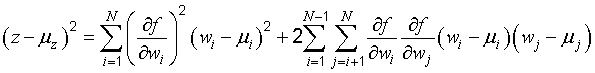(E.2b)
The expectation of the squared deviation (z − μz)2 is the variance of z, that is, E[(z − μz)2] = σ2z, and thus Equation (E.2b) leads to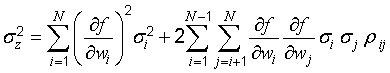(E.3)

In this expression, σ2i = E[(wi − μi)2] is the variance of wi and ρij = υ(wiwj)(σ2iσ2j)1/2 is the correlation coefficient of wi and wj, where υ(wiwj) = E[(wi − μi)(wj − μj)] is the covariance of wi and wj.

NOTE 1   σ2z and σ2i are, respectively, the central moments of order 2 (see C.2.13 and C.2.22) of the probability distributions of z and wi. A probability distribution may be completely characterized by its expectation, variance, and higher‑order central moments.

NOTE 2   Equation (13) in 5.2.2 [together with Equation (15)], which is used to calculate combined standard uncertainty, is identical to Equation (E.3) except that Equation (13) is expressed in terms of estimates of the variances, standard deviations, and correlation coefficients.

E.3.2   In the traditional terminology, Equation (E.3) is often called the “general law of error propagation”, an appellation that is better applied to an expression of the form Δz = ∑Ni = 1(∂fwiwi, where Δz is the change in z due to (small) changes Δwi in the wi [see Equation (E.8)]. In fact, it is appropriate to call Equation (E.3) the law of propagation of uncertainty as is done in this Guide because it shows how the uncertainties of the input quantities wi, taken equal to the standard deviations of the probability distributions of the wi, combine to give the uncertainty of the output quantity z if that uncertainty is taken equal to the standard deviation of the probability distribution of z.

E.3.3   Equation (E.3) also applies to the propagation of multiples of standard deviations, for if each standard deviation σi is replaced by a multiple i, with the same k for each σi, the standard deviation of the output quantity z is replaced by z. However, it does not apply to the propagation of confidence intervals. If each σi is replaced with a quantity δi that defines an interval corresponding to a given level of confidence p, the resulting quantity for z, δz, will not define an interval corresponding to the same value of p unless all of the wi are described by normal distributions. No such assumptions regarding the normality of the probability distributions of the quantities wi are implied in Equation (E.3). More specifically, if in Equation (10) in 5.1.2 each standard uncertainty u(xi) is evaluated from independent repeated observations and multiplied by the t‑factor appropriate for its degrees of freedom for a particular value of p (say p = 95 percent), the uncertainty of the estimate y will not define an interval corresponding to that value of p (see G.3 and G.4).

NOTE   The requirement of normality when propagating confidence intervals using Equation (E.3) may be one of the reasons for the historic separation of the components of uncertainty derived from repeated observations, which were assumed to be normally distributed, from those that were evaluated simply as upper and lower bounds.

E.3.4   Consider the following example: z depends on only one input quantity w, z = f(w), where w is estimated by averaging n values wk of w; these n values are obtained from n independent repeated observations qk of a random variable q; and wk and qk are related by(E.4)

Here α is a constant “systematic” offset or shift common to each observation, and β is a common scale factor. The offset and the scale factor, although fixed during the course of the observations, are assumed to be characterized by a priori probability distributions, with α and β the best estimates of the expectations of these distributions.

The best estimate of w is the arithmetic mean or average w‾‾ obtained from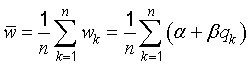(E.5)

The quantity z is then estimated by f(w‾‾ ) = f(αβq1q2, ..., qn) and the estimate u2(z) of its variance σ2(z) is obtained from Equation (E.3). If for simplicity it is assumed that z = w so that the best estimate of z is z = f(w‾‾ ) = w‾‾, then the estimate u2(z) can be readily found. Noting from Equation (E.5) thatanddenoting the estimated variances of α and β by u2(α) and u2(β), respectively, and assuming that the individual observations are uncorrelated, one finds from Equation (E.3)(E.6)
where s2(qk) is the experimental variance of the observations qk calculated according to Equation (4) in 4.2.2, and s2(qk)n = s2(q) is the experimental variance of the mean q [Equation (5) in 4.2.3].

E.3.5   In the traditional terminology, the third term on the right‑hand side of Equation (E.6) is called a “random” contribution to the estimated variance u2(z) because it normally decreases as the number of observations n increases, while the first two terms are called “systematic” contributions because they do not depend on n.

Of more significance, in some traditional treatments of measurement uncertainty, Equation (E.6) is questioned because no distinction is made between uncertainties arising from systematic effects and those arising from random effects. In particular, combining variances obtained from a priori probability distributions with those obtained from frequency‑based distributions is deprecated because the concept of probability is considered to be applicable only to events that can be repeated a large number of times under essentially the same conditions, with the probability p of an event (0 ≤ p ≤ 1) indicating the relative frequency with which the event will occur.

In contrast to this frequency‑based point of view of probability, an equally valid viewpoint is that probability is a measure of the degree of belief that an event will occur [13, 14]. For example, suppose one has a chance of winning a small sum of money D and one is a rational bettor. One's degree of belief in event A occurring is p = 0,5 if one is indifferent to these two betting choices:

1. receiving D if event A occurs but nothing if it does not occur;
2. receiving D if event A does not occur but nothing if it does occur.

Recommendation INC‑1 (1980) upon which this Guide rests implicitly adopts such a viewpoint of probability since it views expressions such as Equation (E.6) as the appropriate way to calculate the combined standard uncertainty of a result of a measurement.

E.3.6   There are three distinct advantages to adopting an interpretation of probability based on degree of belief, the standard deviation (standard uncertainty), and the law of propagation of uncertainty [Equation (E.3)] as the basis for evaluating and expressing uncertainty in measurement, as has been done in this Guide:

1. the law of propagation of uncertainty allows the combined standard uncertainty of one result to be readily incorporated in the evaluation of the combined standard uncertainty of another result in which the first is used;
2. the combined standard uncertainty can serve as the basis for calculating intervals that correspond in a realistic way to their required levels of confidence; and
3. it is unnecessary to classify components as “random” or “systematic” (or in any other manner) when evaluating uncertainty because all components of uncertainty are treated in the same way.

Benefit c. is highly advantageous because such categorization is frequently a source of confusion; an uncertainty component is not either “random” or “systematic”. Its nature is conditioned by the use made of the corresponding quantity, or more formally, by the context in which the quantity appears in the mathematical model that describes the measurement. Thus, when its corresponding quantity is used in a different context, a “random” component may become a “systematic” component, and vice versa.

E.3.7   For the reason given in c. above, Recommendation INC‑1 (1980) does not classify components of uncertainty as either “random” or “systematic”. In fact, as far as the calculation of the combined standard uncertainty of a measurement result is concerned, there is no need to classify uncertainty components and thus no real need for any classificational scheme. Nonetheless, since convenient labels can sometimes be helpful in the communication and discussion of ideas, Recommendation INC‑1 (1980) does provide a scheme for classifying the two distinct methods by which uncertainty components may be evaluated, “A” and “B” (see 0.7, 2.3.2, and 2.3.3).

Classifying the methods used to evaluate uncertainty components avoids the principal problem associated with classifying the components themselves, namely, the dependence of the classification of a component on how the corresponding quantity is used. However, classifying the methods rather than the components does not preclude gathering the individual components evaluated by the two methods into specific groups for a particular purpose in a given measurement, for example, when comparing the experimentally observed and theoretically predicted variability of the output values of a complex measurement system (see 3.4.3).

# E.4   Standard deviations as measures of uncertainty

E.4.1   Equation (E.3) requires that no matter how the uncertainty of the estimate of an input quantity is obtained, it must be evaluated as a standard uncertainty, that is, as an estimated standard deviation. If some “safe” alternative is evaluated instead, it cannot be used in Equation (E.3). In particular, if the “maximum error bound” (the largest conceivable deviation from the putative best estimate) is used in Equation (E.3), the resulting uncertainty will have an ill‑defined meaning and will be unusable by anyone wishing to incorporate it into subsequent calculations of the uncertainties of other quantities (see E.3.3).

E.4.2   When the standard uncertainty of an input quantity cannot be evaluated by an analysis of the results of an adequate number of repeated observations, a probability distribution must be adopted based on knowledge that is much less extensive than might be desirable. That does not, however, make the distribution invalid or unreal; like all probability distributions, it is an expression of what knowledge exists.

E.4.3   Evaluations based on repeated observations are not necessarily superior to those obtained by other means. Consider s(q), the experimental standard deviation of the mean of n independent observations qk of a normally distributed random variable q [see Equation (5) in 4.2.3]. The quantity s(q) is a statistic (see C.2.23) that estimates σ(q), the standard deviation of the probability distribution of q, that is, the standard deviation of the distribution of the values of q that would be obtained if the measurement were repeated an infinite number of times. The variance σ2[s(q)] of s(q) is given, approximately, by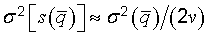(E.7)
where v = n − 1 is the degrees of freedom of s(q) (see G.3.3). Thus the relative standard deviation of s(q), which is given by the ratio σ[s(q)]σ(q) and which can be taken as a measure of the relative uncertainty of s(q), is approximately [2(n − 1)]−1/2. This “uncertainty of the uncertainty” of q, which arises from the purely statistical reason of limited sampling, can be surprisingly large; for n = 10 observations it is 24 percent. This and other values are given in Table E.1, which shows that the standard deviation of a statistically estimated standard deviation is not negligible for practical values of n. One may therefore conclude that Type A evaluations of standard uncertainty are not necessarily more reliable than Type B evaluations, and that in many practical measurement situations where the number of observations is limited, the components obtained from Type B evaluations may be better known than the components obtained from Type A evaluations.
Table E.1 — σ[s(q)]σ(q), the standard deviation of the experimental standard deviation of the mean q of n independent observations of a normally distributed random variable q, relative to the standard deviation of that mean(a) (b)
Number of observations σ[s(q)]σ(q)
n (percent)

(a)   The values given have been calculated from the exact expression for σ[s(q)]σ(q), not the approximate expression [2(n − 1)]−1/2.

(b)   In the expression σ[s(q)]σ(q), the denominator σ(q) is the expectation E [Sn‾‾‾‾] and the numerator σ[s(q)] is the square root of the variance V [Sn‾‾‾‾], where S denotes a random variable equal to the standard deviation of n independent random variables X1, ..., Xn, each having a normal distribution with mean value μ and variance σ2: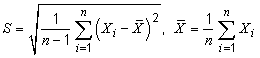The expectation and variance of S are given by: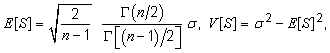where Γ(x) is the gamma function. Note that E [S] < σ for a finite number n.

2 76
3 52
4 42
5 36
10 24
20 16
30 13
50 10

E.4.4   It has been argued that, whereas the uncertainties associated with the application of a particular method of measurement are statistical parameters characterizing random variables, there are instances of a “truly systematic effect” whose uncertainty must be treated differently. An example is an offset having an unknown fixed value that is the same for every determination by the method due to a possible imperfection in the very principle of the method itself or one of its underlying assumptions. But if the possibility of such an offset is acknowledged to exist and its magnitude is believed to be possibly significant, then it can be described by a probability distribution, however simply constructed, based on the knowledge that led to the conclusion that it could exist and be significant. Thus, if one considers probability to be a measure of the degree of belief that an event will occur, the contribution of such a systematic effect can be included in the combined standard uncertainty of a measurement result by evaluating it as a standard uncertainty of an a priori probability distribution and treating it in the same manner as any other standard uncertainty of an input quantity.

EXAMPLE   The specification of a particular measurement procedure requires that a certain input quantity be calculated from a specific power‑series expansion whose higher‑order terms are inexactly known. The systematic effect due to not being able to treat these terms exactly leads to an unknown fixed offset that cannot be experimentally sampled by repetitions of the procedure. Thus the uncertainty associated with the effect cannot be evaluated and included in the uncertainty of the final measurement result if a frequency‑based interpretation of probability is strictly followed. However, interpreting probability on the basis of degree of belief allows the uncertainty characterizing the effect to be evaluated from an a priori probability distribution (derived from the available knowledge concerning the inexactly known terms) and to be included in the calculation of the combined standard uncertainty of the measurement result like any other uncertainty.

# E.5   A comparison of two views of uncertainty

E.5.1   The focus of this Guide is on the measurement result and its evaluated uncertainty rather than on the unknowable quantities “true” value and error (see Annex D). By taking the operational views that the result of a measurement is simply the value attributed to the measurand and that the uncertainty of that result is a measure of the dispersion of the values that could reasonably be attributed to the measurand, this Guide in effect uncouples the often confusing connection between uncertainty and the unknowable quantities “true” value and error.

E.5.2   This connection may be understood by interpreting the derivation of Equation (E.3), the law of propagation of uncertainty, from the standpoint of “true” value and error. In this case, μi is viewed as the unknown, unique “true” value of input quantity wi and each wi is assumed to be related to its “true” value μi by wi = μi + εi, where εi is the error in wi. The expectation of the probability distribution of each εi is assumed to be zero, E(εi) = 0, with variance E(ε2i) = σ2i. Equation (E.1) becomes then(E.8)

where εz = z − μz is the error in z and μz is the “true” value of z. If one then takes the expectation of the square of εz, one obtains an equation identical in form to Equation (E.3) but in which σ2z = E(ε2z) is the variance of εz and ρij = υ(εiεj)(σ2i  σ2j)1/2 is the correlation coefficient of εi and εj, where υ(εiεj) = E(εi εj) is the covariance of εi and εj. The variances and correlation coefficients are thus associated with the errors of the input quantities rather than with the input quantities themselves.

NOTE   It is assumed that probability is viewed as a measure of the degree of belief that an event will occur, implying that a systematic error may be treated in the same way as a random error and that εi represents either kind.

E.5.3   In practice, the difference in point of view does not lead to a difference in the numerical value of the measurement result or of the uncertainty assigned to that result.

First, in both cases, the best available estimates of the input quantities wi are used to obtain the best estimate of z from the function f; it makes no difference in the calculations if the best estimates are viewed as the values most likely to be attributed to the quantities in question or the best estimates of their “true” values.

Second, because εi = wi − μi, and because the μi represent unique, fixed values and hence have no uncertainty, the variances and standard deviations of the εi and wi are identical. This means that in both cases, the standard uncertainties used as the estimates of the standard deviations σi to obtain the combined standard uncertainty of the measurement result are identical and will yield the same numerical value for that uncertainty. Again, it makes no difference in the calculations if a standard uncertainty is viewed as a measure of the dispersion of the probability distribution of an input quantity or as a measure of the dispersion of the probability distribution of the error of that quantity.

NOTE   If the assumption of the note of E.5.2 had not been made, then the discussion of this subclause would not apply unless all of the estimates of the input quantities and the uncertainties of those estimates were obtained from the statistical analysis of repeated observations, that is, from Type A evaluations.

E.5.4   While the approach based on “true” value and error yields the same numerical results as the approach taken in this Guide (provided that the assumption of the note of E.5.2 is made), this Guide's concept of uncertainty eliminates the confusion between error and uncertainty (see Annex D). Indeed, this Guide's operational approach, wherein the focus is on the observed (or estimated) value of a quantity and the observed (or estimated) variability of that value, makes any mention of error entirely unnecessary.